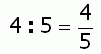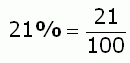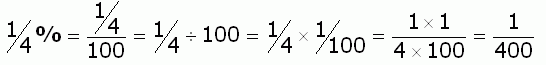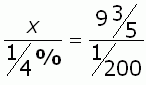SEARCH HOMEMath Central Quandaries & QueriesQuestion from Charon, a student: Example:                      x : 1/4% :: 9 3/5 : 1/200Hi Charon.

Ratios are fractions, and so are percentages.

For example,And when you have a fraction of a percentage, then you have a fraction of a fraction, so you can think of it as multiplying by the inverse of the reciprocal of the denominator, like this:I presume by the double colon, you mean equals. So the question is really asking this:Try simplifying the fractions first, then isolate x, then "invert and multiply" to find the answer.

Cheers,
Stephen La Rocque.Math Central is supported by the University of Regina and The Pacific Institute for the Mathematical Sciences.